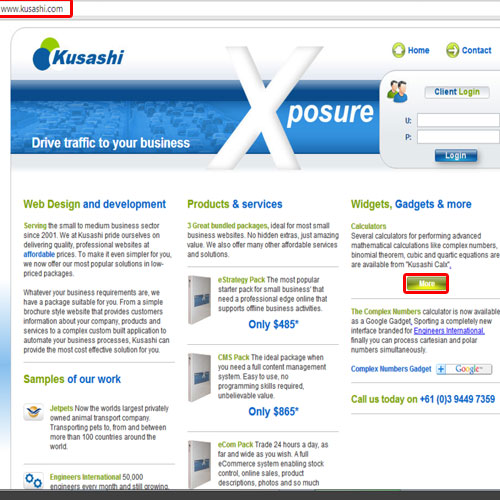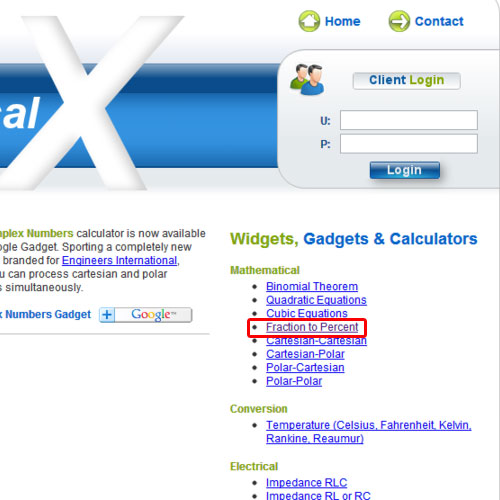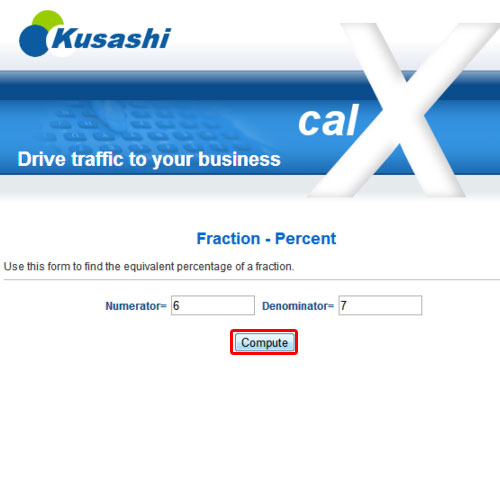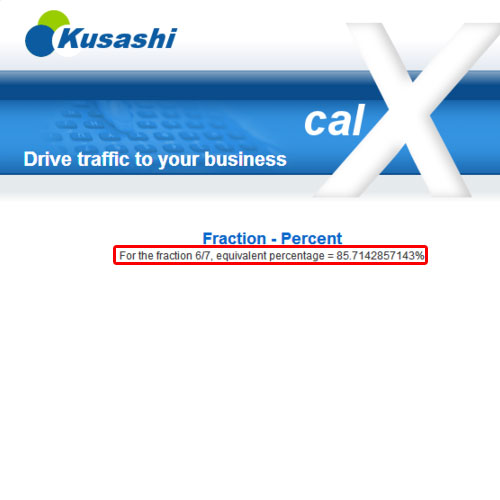## How to Use Fraction to Percent Calculator

In this video, we will show you how to use a fraction to percent calculator. A fraction comprises of a numerator and denominator. This calculator computes what percentage the numerator forms of the denominator. We will teach you how to work with fractions and percents.

Step 1 – Go to widgets, gadgets & more

First of all, open any web browser of your choice and go to kusashi.com. Once the web page opens, go to the “widgets, gadgets & more” section and click on the more button.Step 2 – Click on the fraction to percent option

As a result, the calculator page will open. Go to the right hand side of the page and from the mathematical category, click on the fraction to percent option. This would allow you to work with fractions and percents.Step 3 – Equivalent percentage of a fraction

A form will open up from where you can find the equivalent percentage of a fraction. Simply fill in the numerator and denominator fields. Once you are done, click on the compute button.Step 4 – Computation Results

For the purpose of this tutorial, we will place 6 as the numerator and 7 as the denominator. The result after computation will be 85.71 percent. What this calculator does is that it computes what percentage the numerator forms of the denominator.
In this manner, you can work with the fraction to percent calculator.